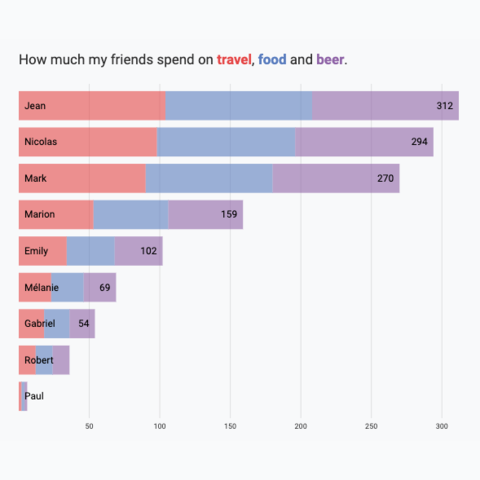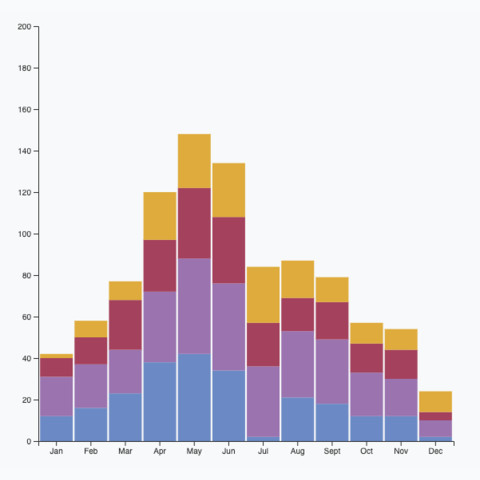# BarplotA barplot displays a numeric value for several groups of a dataset using rectangles. This page is a step-by-step guide on how to build your own barplot for the web, using React and D3.js.

It starts with very basic concepts like data structure, scales and svg rectangle rendering. It then shows how to add interactivity to the chart with hover effects. Last but not least it explains how to build variations like the stacked barplot.

## The Data

The dataset required to build a barplot is usually an array where each item is an object providing the `name` and the `value` of the group.

Here is a minimal example

``````const data = [
{name:"Mark", value: 90},
{name:"Robert", value: 12},
{name:"Emily", value: 34},
{name:"Marion", value: 53},
{name:"Nicolas", value: 98},
]``````

Note: if your data is in `.csv` format, you can translate it thanks to the `d3.csv()` function as suggested here.

## Component skeleton

The goal here is to create a `Barplot` component that will be stored in a `Barplot.tsx` file. This component requires 3 props to render: a `width`, a `height`, and some `data`.

The shape of the `data` is described above. The `width` and `height` will be used to rendering a `svg` element in the DOM, in which we will insert the barplot.

To put it in a nutshell, that's the skeleton of our `Barplot` component:

``````import * as d3 from "d3"; // we will need d3.js

type BarplotProps = {
width: number;
height: number;
data: { name: string; y: number }[];
};

export const Barplot = ({ width, height, data }: BarplotProps) => {

// do some stuff with d3
// compute all the <rect>

return (
<div>
<svg width={width} height={height}>
// render all the <rect>
</svg>
</div>
);
};``````

It's fundamental to understand that with this code organization, d3.js will be used to prepare the svg `circle`, but it's react that will render them in the `return()` statement. We won't use d3 methods like `append` that you can find in usual d3.js examples.

## Scales

A scale is a function that transforms a dimension (like our `value` or our group `name`) in a position in pixels.

Building a barplot requires 2 scales of 2 kinds. The first will transform the group `value` in a bar length. The second will transform the group `name` in a position.

### → Linear scale for the bar length

D3.js comes with a handful set of predefined scales. `scaleLinear` is what we need for the bar length. Here is a quick overview on how to build and use a linear scale:

``````const scale = d3.scaleLinear()
.domain([0, 10]) // data goes from 0 to 10
.range([0, 200]); // axis goes from 0 to 200

scale(0); // 0 -> item with a value of 0 will have a bar of length 0
scale(5); // 100 -> bar of length 100
scale(10); // 200 -> bar of length 200``````

Since we are building a horizontal barplot here, this scale will be used by the X axis.

To dig more into d3 scales, visit this dedicated page. It's a crucial concept that will be used everywhere in this website.

### → Band scale for the group position

A band scale will be used to control the position of each rectangle on the Y axis. It is computed with the `scaleBand()` function of d3.js. It attributes a band of pixels to each group.

For instance, calling the band scale with `yScale("A")` will return `0`, and `yScale.bandwidth()` will return the width of the band (e.g. `11px`).

Note: the `padding` argument controls the space between bars.

``````const yScale = d3
.scaleBand()
.range([0, boundsHeight])
.domain(allGroups)

// yScale("A") -> 0
// yScale.bandwidth() -> 11``````

## Basic barplot

We now have all the ingredients to build a basic barplot with react, all being pretty close to the d3-only examples.

For each item in the dataset, create a SVG `rect` element. Its vertical position can be retrieved from the group `name` thanks to the band scale. It's size is retrieved using the `xScale` and its `value`.

Note that using the same amount of information it is straightforward to add a label for the name and one for the value.

Most basic barplot built with d3.js for scales, and react for rendering

## Responsive Barplot with react

The component above is not responsive. It expects 2 props called `width` and `height` and will render a Barplot of those dimensions.

Making the Barplot responsive requires adding a wrapper component that gets the dimension of the parent `div`, and listening to a potential dimension change. This is possible thanks to a hook called `useDimensions` that will do the job for us.

`useDimensions`: a hook to make your viz responsive
``````export const useDimensions = (targetRef: React.RefObject<HTMLDivElement>) => {

const getDimensions = () => {
return {
width: targetRef.current ? targetRef.current.offsetWidth : 0,
height: targetRef.current ? targetRef.current.offsetHeight : 0
};
};

const [dimensions, setDimensions] = useState(getDimensions);

const handleResize = () => {
setDimensions(getDimensions());
};

useEffect(() => {
return () => window.removeEventListener("resize", handleResize);
}, []);

useLayoutEffect(() => {
handleResize();
}, []);

return dimensions;
}``````

I'm in the process of writing a complete blog post on the topic. Subscribe to the project to know when it's ready.

## Transition

When the dataset updates, it adds a nice touch to smoothly animate the transition. In the example below, changing the dataset will update the bar sizes and their positions on the Y axis to keep the ranking accurate.

Animation is a complicated topic in dataviz. We have to deal with updates (an element changes its features), enter (a new element appears) and exit (an element is not present anymore) patterns.

I suggest to rely on the `react-spring` library to help here. Please check this dedicated blogpost to get explanations about the code of this example.

Most basic barplot built with d3.js for scales, and react for rendering

## Stacking

A stacked barplot is a variation of a barplot where an additional level of grouping is represented. Each bar represent the value of a `group`, for instance how much each my friend spent in the last month. Each bar is then subdivided, each part representing the value of a `subgroup`, for instance the category of expense.

D3 comes with a very handy `stack()` function. The 2 tutorials below explain how this function works, and how to use it to render a clean stacked barplot.Horizontal Stacked BarplotRepresent group and subgroup values by stacking the dataVertical Stacked BarplotRepresent group and subgroup values by stacking the data

## Vertical barplot

The vertical option is less common since it makes is much harder to read the labels. But if you really need it, it is just a matter of swaping the X and Y axes of the previous example.

This example will be publish soon, please subscribe below if you want to be notified.

## Hover effect

This example will be publish soon, please subscribe to the newsletter if you want to be notified.

## Contact

👋 Hey, I'm Yan and I'm currently working on this project!

Feedback is welcome ❤️. You can fill an issue on Github, drop me a message on Twitter, or even send me an email pasting `yan.holtz.data` with `gmail.com`. You can also subscribe to the newsletter to know when I publish more content!